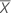# Methods and formulas for 1-Sample Equivalence Test

## Difference

### Notation

TermDescription
DDifferenceTest mean

## Standard error of the difference (SE)

### Notation

TermDescription
sStandard deviation of the observations
nNumber of observations

## Equivalence limits

Let k1and k2 be the values that you specify for the lower limit and the upper limit, respectively. By default, the lower equivalence limit, δ1, is given by: and the upper equivalence limit, δ2, is given by:
However, if you select the option to multiply your limits by the target value, then the limits are given by:

## Degrees of freedom (DF)

### Notation

TermDescription
vDegrees of freedom
nNumber of observations

## Confidence interval for the difference

### 100(1 – α)% CI

By default, Minitab uses the following formula to calculate the 100(1 – α)% confidence interval (CI) for the difference:

CI = [min(C, Dl), max(C, Du)]

where:

### 100(1 – 2α)% CI

If you select the option to use the 100(1 – 2 α)% CI, then the CI is given by the following formula:

CI = [Dl, Du]

### One-sided intervals

For a hypotheses of Test mean > target, or Test mean - target > lower limit, the 100(1 – α)% lower bound is equal to DL.

For a hypothesis of Test mean < target, or Test mean - target < upper limit, the 100(1 – α)% upper bound is equal to DU.

### Notation

TermDescription
DDifference between the mean of the test sample and the target value
SEStandard error
δ1Lower equivalence limit
δ2Upper equivalence limit
vDegrees of freedom
αSignificance level for the test
t1 – α, vUpper 1 – α critical value for a t-distribution with v degrees of freedom

## T-values

Let t 1 be the t-value for the hypothesis,, and let t 2 be the t-value for the hypothesis,, whereis the difference between the mean of the test population and the target value. By default, the t-values are calculated as follows:

For a hypothesis of Test mean > target, δ1= 0.

For a hypothesis of Test mean < target, δ2= 0.

### Notation

TermDescription
DDifference between the mean of the test sample and the target value
SEStandard error of the difference
δ1Lower equivalence limit
δ2Upper equivalence limit

## P-values

The probability, PH0, for each null hypothesis (H0) is given by the following:

H0 P-Value### Notation

TermDescriptionUnknown difference between the mean of the test population and the target value
δ1Lower equivalence limit
δ2Upper equivalence limit
vDegrees of freedom
Tt distribution with v degrees of freedom
t1The t-value for the hypothesist2The t- value for the hypothesis###### Note

For information on how the t-values are calculated, see the section on t-values.

By using this site you agree to the use of cookies for analytics and personalized content.  Read our policy